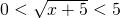In this section, radical equations that need to solved are discussed. The strategy is relatively simple: isolate the radical on one side of the equation and all variables will remain on the other side. Once this is done, square, cube, or raise each side to a power that removes the radical. For example: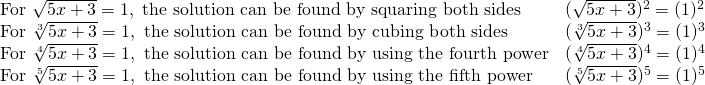Once the radical is removed, then solve the resulting equation. Consider how this strategy is used in the following example.

Example 10.1.1

Solve for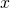in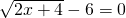.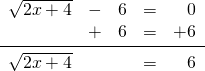This leaves: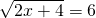Squaring both sides: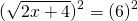This results in: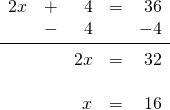This same strategy works for equations having indices larger than 2.

Example 10.1.2

Solve forin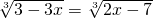.

For this problem, there are two radicals equalling each other, and all that is required is to cube each to cancel the radicals out.

Because there is an odd index, there will have two solutions to this equation: one positive and one negative.

## Positive Solution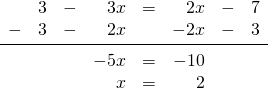## Negative SolutionThe strategy used above to isolate and solve for the radicals works the same for radicals in inequalities, except that you will now have to square, cube or use a larger power on each of the terms. For example:

Example 10.1.3

Solve forin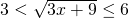.

For this problem, there are three terms to square out.

This looks like: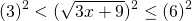Which results in: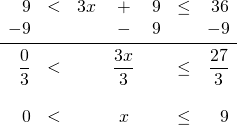For all cases of radical equations, check answers to see if they work. There may be variations of these radical equations in higher levels of math, but the strategy will always be similar in that you will always work to square the radicals out.

# Questions

1.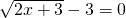2.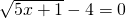3.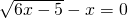4.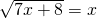5.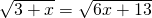6.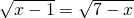7.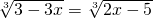8.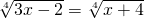9.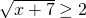10.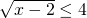11.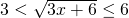12.